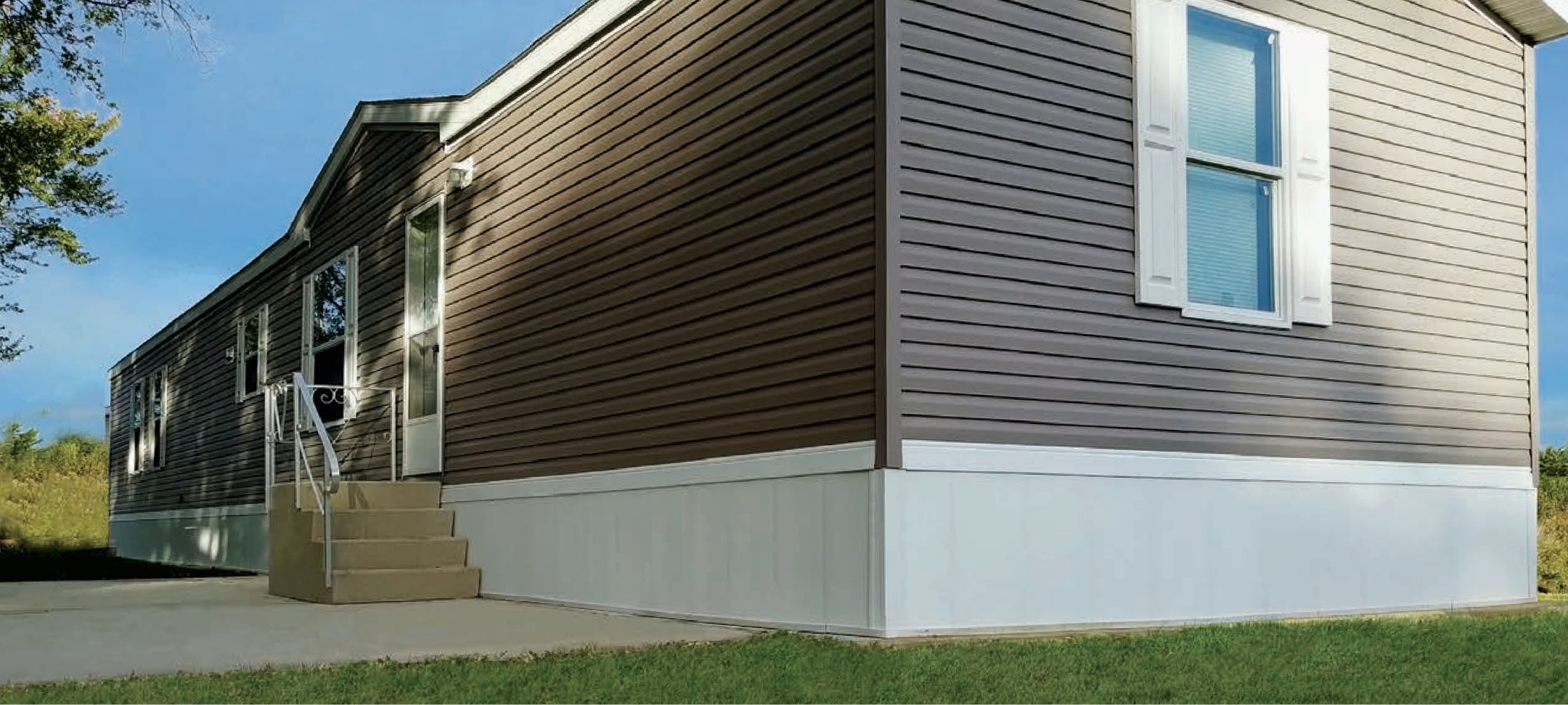# Mobile Home Skirting Kit Configurator

## Build-Your-Own Skirting Kit

Calculate Home PerimeterMeasure the length and width of your home and enter into appropriate fields below to calculate perimeter*For additional coverage (decks, patios, etc.), simply measure the length that the given area(s) extend from your home. If you have multiple such areas, simply provide the sum total of the measurements.
Calculate Average Height of HomeMeasure the height of each corner (4) and the height of each midpoint of each long side (2), rounding up to the nearest inch, to calculate average height
• Calculate avg height
• I know my avg height

#### Height Measurements

Prices based on home measurements provided in previous sections:

• Perimeter Measurement:
• Average Height: